### Home > CAAC > Chapter 8 > Lesson 8.2.4 > Problem8-80

8-80.
1. Calculate the expressions below with a scientific calculator. Homework Help ✎

1.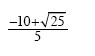2.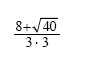3.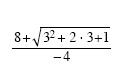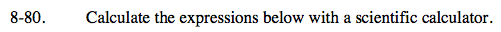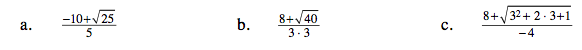Calculate the square root, then simplify the rest of the expression.

$\frac{-10+5}{5}=\frac{-5}{5}$

$\frac{-5}{5}=-1$

Calculate the square root and everything in the denominator before simplifying the rest of the expression.

Calculate everything inside the square root before simplifying.